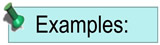Factoring by Grouping MathBitsNotebook.com Terms of Use   Contact Person: Donna RobertsThere are many different applications of factoring by grouping.
We will be examining factoring by grouping as it applies to trinomials of the form ax2 + bx + c.

Notice: (x + 4)(x + 6) = x2 + 4x + 6x + 24 = x2 + 10x + 24

Whenever you multipy two binomials, you create two "middle" terms, which in many cases are "like" terms. It is the creation of these "middle" terms which becomes the focus of the process of factoring by grouping for a trinomial. This method is often called "split the middle" since it endeavors to create two "middle" terms which will make the factoring process easier.

Factoring by grouping is a valuable strategy. It can be used on a variety of problems and is popular in college mathematics textbooks. You will definitely see this process again.Process of Factoring by Grouping:
 To factor a trinomial of the form ax2 + bx + c: Factor: 8x2 + 26x + 15 1. Always check for any common factors before you begin. It will make finding the solution easier. There are no common factors in this trinomial. 2. Find a • c (referred to as the Master Product). a • c = 8 • 15 = 120 3. Find two new factors of a•c (120) that add up to b (+26). Let the two factors be m and n. Then m • n = 120 and m + n = 26. The new factors are m = 20 and n = 6. New factors: 20 • 6 = 120 20 + 6 = 26 4. Split the middle term into two terms using the sum of the two new factors, including the proper signs.The order of the middle terms does not matter. They will give the correct answer in either order. 8x2 + 20x + 6x + 15 order of middle terms does not matter 5. Group the four terms to form two pairs. Be careful of the signs. (See example below for dealing with grouping a negative middle term.) (8x2 + 20x) + (6x + 15) 6. Factor each pair by finding the common factors. 4x(2x + 5) + 3(2x + 5) 7. Factor out the common (shared) binomial. (2x + 5)(4x + 3)

 In plain English: . To get the "factoring by grouping" process started, you are looking for two numbers that multiply to ac, and whose sum is equal to b. These two numbers will help form the new "middle terms", which will allow for grouping and factoring to arrive at the answer.It is important to be careful when dealing with negative symbols.1. Factor: x2 + x - 12
Yes, factoring by grouping can work on even simple trinomial factoring problems with a leading coefficient of one.2. Factor: 2x2 - 13x + 21
Factoring by grouping is more widely used on more difficult problems with a leading coefficient other than one.
a • c = 1 • (-12) = -12
a • c = 2 • 21 = 42
 (-3) • 4 = -12 (-3) + 4 = +1 These are the new factors that multiply to a•c (-12) and add to b (+1).
 (-6) • (-7) = 42 (-6) + (-7) = -13 These are the new factors that multiply to a•c (42) and add to b (-13).
x2 + 4x - 3x - 12
2x2 - 6x - 7x + 21
(x2 + 4x) - 1(3x + 12)
The safest way to "group" when the second middle term is negative is to factor out a -1 from the second pairing. Less errors are made with this approach.
(2x2 - 6x) - 1(7x - 21)
The safest way to "group" when the second middle term is negative is to factor out a -1 from the second pairing. Less errors are made with this approach.
x(x + 4) -1•3(x + 4)
x(x + 4)
- 3(x + 4)
2x(x - 3) - 1•7(x - 3)
2x(x - 3) - 7(x - 3)
(x + 4)(x - 3) Answer
(x - 3)(2x - 7) Answer

At the top of this page, it was stated that multiplying binomials often creates two middle terms that are "like" terms. But, of course, not ALL binomial products produce "like" middle terms.

Consider: (a + b)(x + y) = ax + ay + bx + by

In this expression, there is no factor (other than 1) that is common to all four terms. There is, however, a common factor of a for the first two terms, and a common factor of b for the last two terms.
So, "factoring by grouping" will be a strategy for dealing with finding the factors of an expression such as ax + ay + bx + by.3. Factor: ax + ay + bx + by4. Factor: 5x3 + 15x2 - 10x - 30 There is no need to create the sum of the "middle" terms as was needed when dealing with the trinomials. Go right to the "grouping" process. Factor out the common factor of 5: 5[x3 + 3x2 - 2x - 6] (ax + ay) + (bx + by) 5[(x3 + 3x2) -1(2x + 6)] a(x + y) + b(x + y) 5[x2(x + 3) -1•2(x + 3)] 5[x2(x + 3) - 2(x + 3)] (x + y)(a + b) 5(x + 3)(x2 - 2)